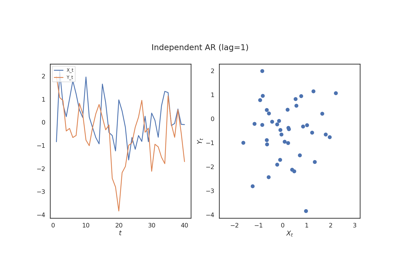# MGCX¶

class hyppo.time_series.MGCX(compute_distance='euclidean', max_lag=0, **kwargs)

Cross Multiscale Graph Correlation (MGCX) test statistic and p-value.

MGCX is an independence test between two (paired) time series of not necessarily equal dimensions. The population parameter is 0 if and only if the time series are independent. It is based upon energy distance between distributions.

Parameters
• compute_distance (str, callable, or None, default: "euclidean") -- A function that computes the distance among the samples within each data matrix. Valid strings for compute_distance are, as defined in sklearn.metrics.pairwise_distances,

• From scikit-learn: ["euclidean", "cityblock", "cosine", "l1", "l2", "manhattan"] See the documentation for scipy.spatial.distance for details on these metrics.

• From scipy.spatial.distance: ["braycurtis", "canberra", "chebyshev", "correlation", "dice", "hamming", "jaccard", "kulsinski", "mahalanobis", "minkowski", "rogerstanimoto", "russellrao", "seuclidean", "sokalmichener", "sokalsneath", "sqeuclidean", "yule"] See the documentation for scipy.spatial.distance for details on these metrics.

Set to None or "precomputed" if x and y are already distance matrices. To call a custom function, either create the distance matrix before-hand or create a function of the form metric(x, **kwargs) where x is the data matrix for which pairwise distances are calculated and **kwargs are extra arguements to send to your custom function.

• max_lag (int, default: 0) -- The maximum number of lags in the past to check dependence between x and the shifted y. Also the M hyperparmeter below.

• **kwargs -- Arbitrary keyword arguments for compute_distance.

Notes

The statistic can be derived as follows 1:

Let $$x$$ and $$y$$ be $$(n, p)$$ and $$(n, q)$$ series respectively, which each contain $$y$$ observations of the series $$(X_t)$$ and $$(Y_t)$$. Similarly, let $$x[j:n]$$ be the $$(n-j, p)$$ last $$n-j$$ observations of $$x$$. Let $$y[0:(n-j)]$$ be the $$(n-j, p)$$ first $$n-j$$ observations of $$y$$. Let $$M$$ be the maximum lag hyperparameter. The cross distance correlation is,

$\mathrm{MGCX}_n (x, y) = \sum_{j=0}^M \frac{n-j}{n} MGC_n (x[j:n], y[0:(n-j)])$

References

1

Ronak Mehta, Jaewon Chung, Cencheng Shen, Ting Xu, and Joshua T. Vogelstein. Independence Testing for Multivariate Time Series. arXiv:1908.06486 [cs, stat], May 2020. arXiv:1908.06486.

Methods Summary

 MGCX.statistic(x, y) Helper function that calculates the MGCX test statistic. MGCX.test(x, y[, reps, workers, random_state]) Calculates the MGCX test statistic and p-value.

MGCX.statistic(x, y)

Helper function that calculates the MGCX test statistic.

Parameters

x,y (ndarray) -- Input data matrices. x and y must have the same number of samples. That is, the shapes must be (n, p) and (n, q) where n is the number of samples and p and q are the number of dimensions. Alternatively, x and y can be distance matrices, where the shapes must both be (n, n).

Returns

• stat (float) -- The computed MGCX statistic.

• opt_lag (int) -- The computed optimal lag.

• opt_scale ((int, int)) -- The computed optimal scale as a pair of two elements.

MGCX.test(x, y, reps=1000, workers=1, random_state=None)

Calculates the MGCX test statistic and p-value.

Parameters
• x,y (ndarray) -- Input data matrices. x and y must have the same number of samples. That is, the shapes must be (n, p) and (n, q) where n is the number of samples and p and q are the number of dimensions. Alternatively, x and y can be distance matrices, where the shapes must both be (n, n).

• reps (int, default: 1000) -- The number of replications used to estimate the null distribution when using the permutation test used to calculate the p-value.

• workers (int, default: 1) -- The number of cores to parallelize the p-value computation over. Supply -1 to use all cores available to the Process.

• auto (bool, default: True) -- Automatically uses fast approximation when n and size of array is greater than 20. If True, and sample size is greater than 20, then hyppo.tools.chi2_approx will be run. Parameters reps and workers are irrelevant in this case. Otherwise, hyppo.tools.perm_test will be run.

Returns

• stat (float) -- The computed MGCX statistic.

• pvalue (float) -- The computed MGCX p-value.

• mgcx_dict (dict) --

Contains additional useful returns containing the following keys:

• opt_lagint

The optimal lag that maximizes the strength of the relationship with respect to lag.

• opt_scale(int, int)

The optimal scale that maximizes the strength of the relationship with respect to scale.

Examples

The optimal scale should be global (n, n) for cases of linear correlation.

>>> import numpy as np
>>> from hyppo.time_series import MGCX
>>> np.random.seed(456)
>>> x = np.arange(7)
>>> y = x
>>> stat, pvalue, mgcx_dict = MGCX().test(x, y, reps = 100)
>>> '%.1f, %.2f, [%d, %d]' % (stat, pvalue, mgcx_dict['opt_scale'],
... mgcx_dict['opt_scale'])
'1.0, 0.05, [7, 7]'


The increasing the max_lag can increase the ability to identify dependence.

>>> import numpy as np
>>> from hyppo.time_series import MGCX
>>> np.random.seed(1234)
>>> x = np.random.permutation(10)
>>> y = np.roll(x, -1)
>>> stat, pvalue, mgcx_dict = MGCX(max_lag=1).test(x, y, reps=1000)
>>> '%.1f, %.2f, %d' % (stat, pvalue, mgcx_dict['opt_lag'])
'1.1, 0.01, 1'


## Examples using hyppo.time_series.MGCX¶